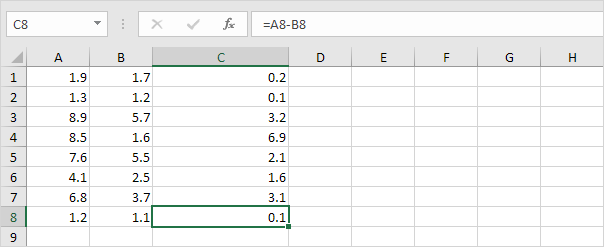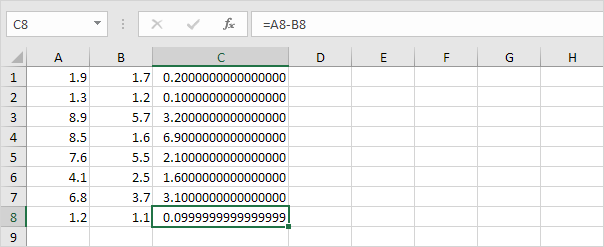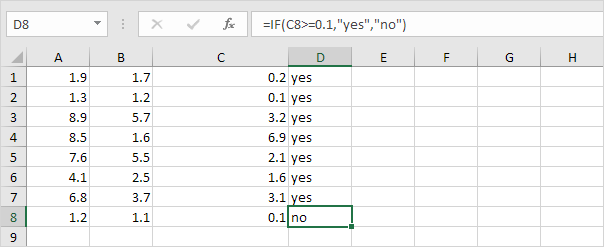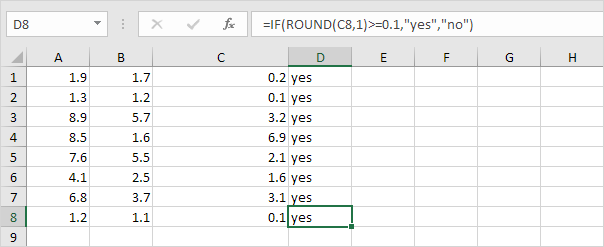## Excel Office

Excel How Tos, Tutorials, Tips & Tricks, Shortcuts

# Floating Point Errors in Excel

Floating point numbers are numbers that follow after a decimal point.

Excel stores and calculates floating point numbers.

On the other hand, excel cannot store numbers with very large floating points, so for the function to work correctly, the floating point numbers will need to be rounded to 5 decimal places. i.e The result of a formula is a very close approximation.

Also See:   How to increase by percentage in Excel

1. For example, take a look at the formulas below. At first glance, everything looks alright.2. However, if we show 16 decimal places, we can see that one result is a very close approximation.You don’t have to worry about floating point errors. They are rare.

3. Even if your worksheet contains a floating point error, in most cases, this causes no problems. However, if you compare the value in cell C8 with another value, the following problem can occur.

Also See:   Understanding Various Paste Options in Excel4. Use the ROUND function to fix this.